﻿ Calculation of a fluid bed - XSim

# Calculation of a fluid bed

Update: March 16, 2018
OpenFOAM 4.x

## Case directory

\$FOAM_TUTORIALS/lagrangian/MPPICFoam/Goldschmidt

## Summary

We perform unsteady calculations of a fluid bed for 5 seconds. The computational domain is a rectangular box with air flowing in vertically from the region "bottom" at 1.875 m/s and flowing out from the region "top". The side walls are assumed to be no-slip walls. And thickness direction walls are assumed to be symmetric walls.Model geometry

The initial positions and properties of the discrete particles are defined in the files "kinematicCloudPositions" and "kinematicCloudProperties" in the directory "constant", respectively. Fluid calculations are performed without using turbulence models.

The meshes are as follows.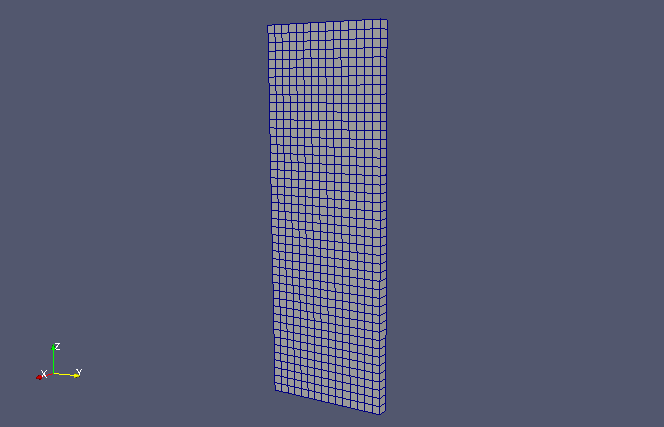Meshes

The calculation result is as follows.

Discrete particles's motion (at 1/4 speed, color represents magnitude of flow velocity)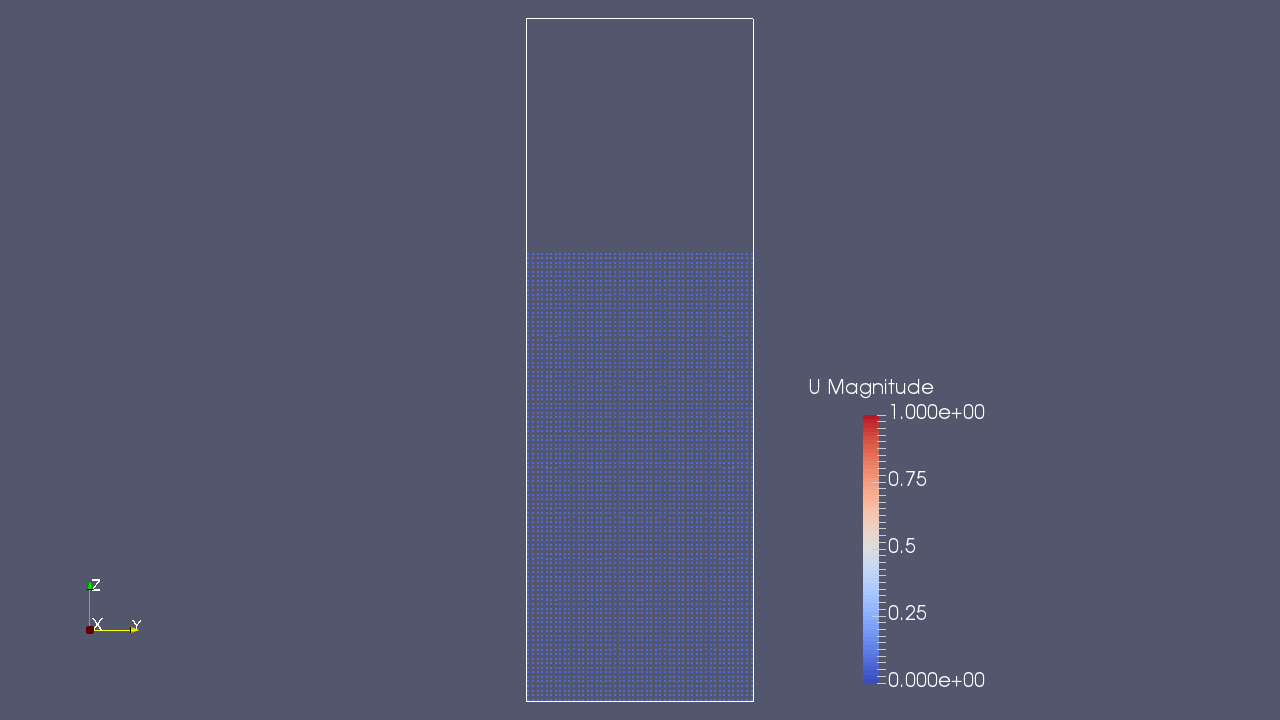Discrete particles and the velocity at 0 sec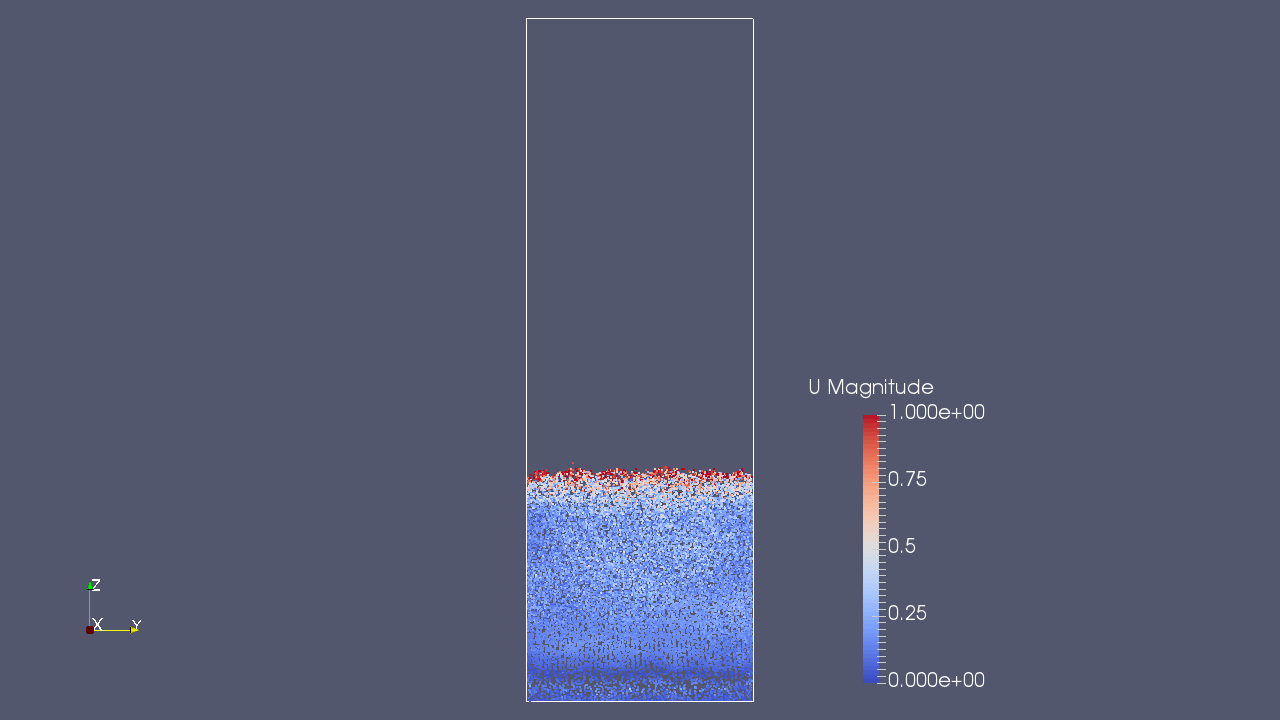Discrete particles and the velocity at 0.25 sec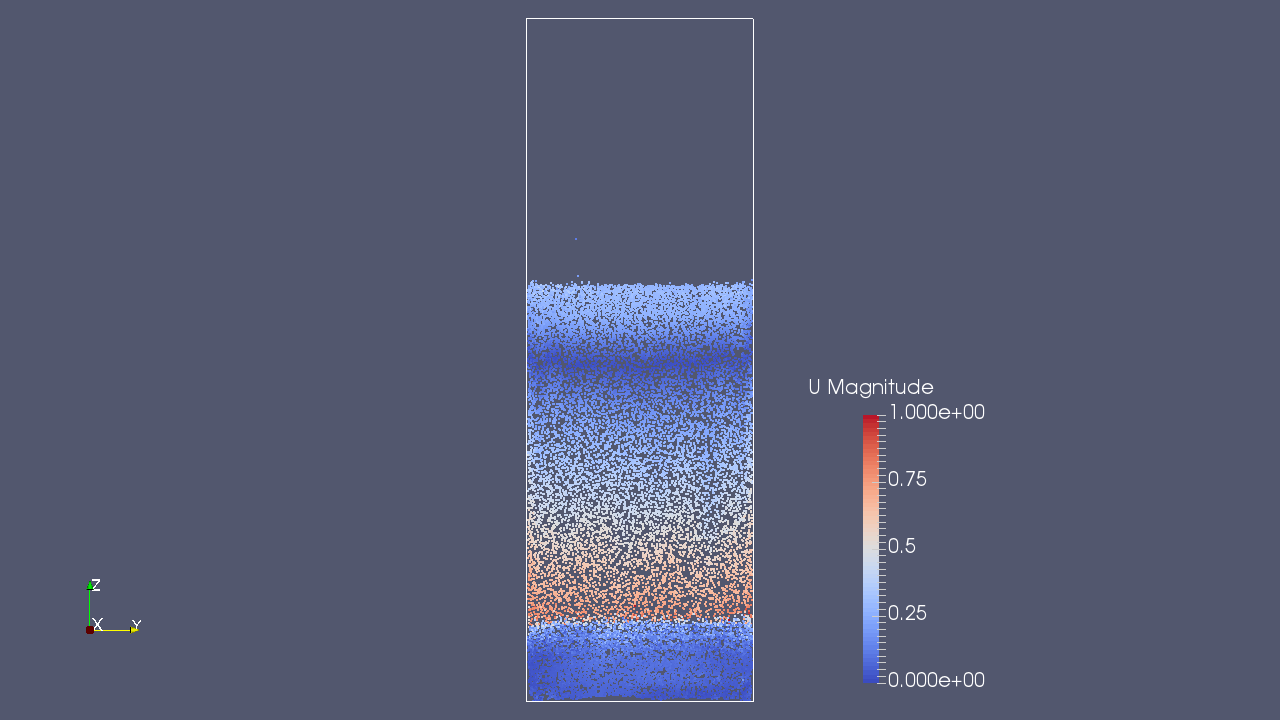Discrete particles and the velocity at 0.5 sec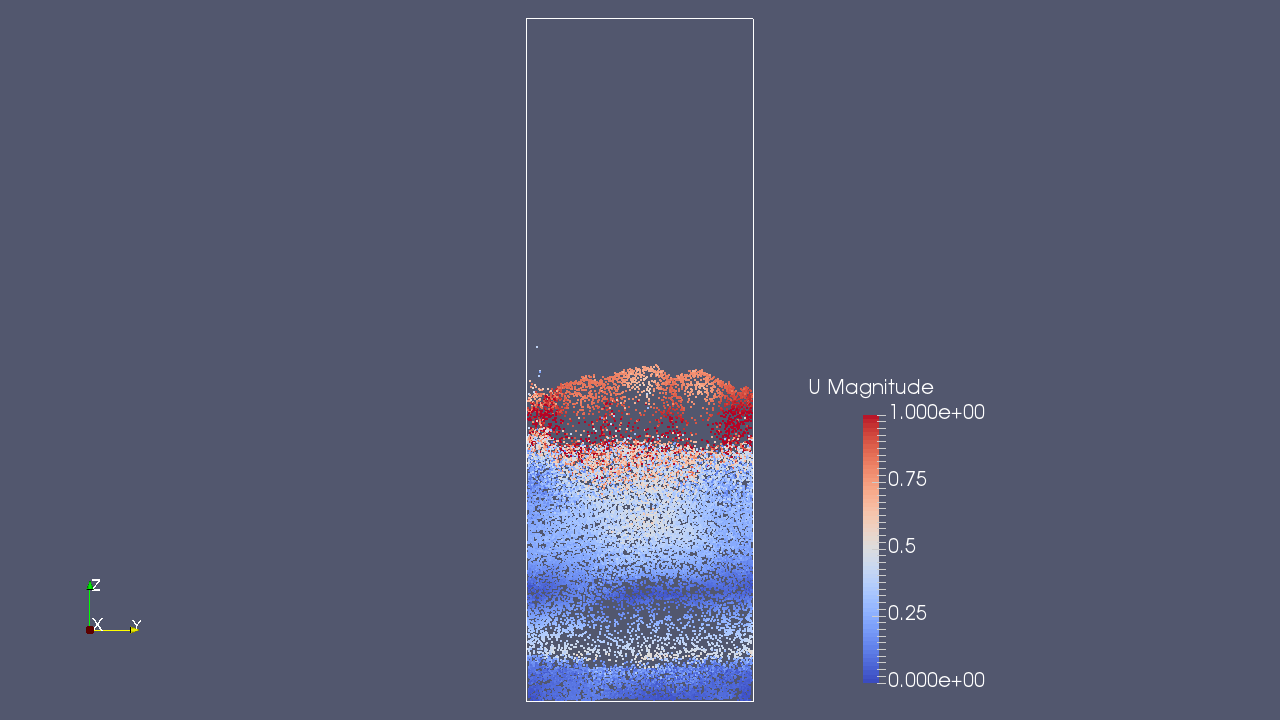Discrete particles and the velocity at 0.75 sec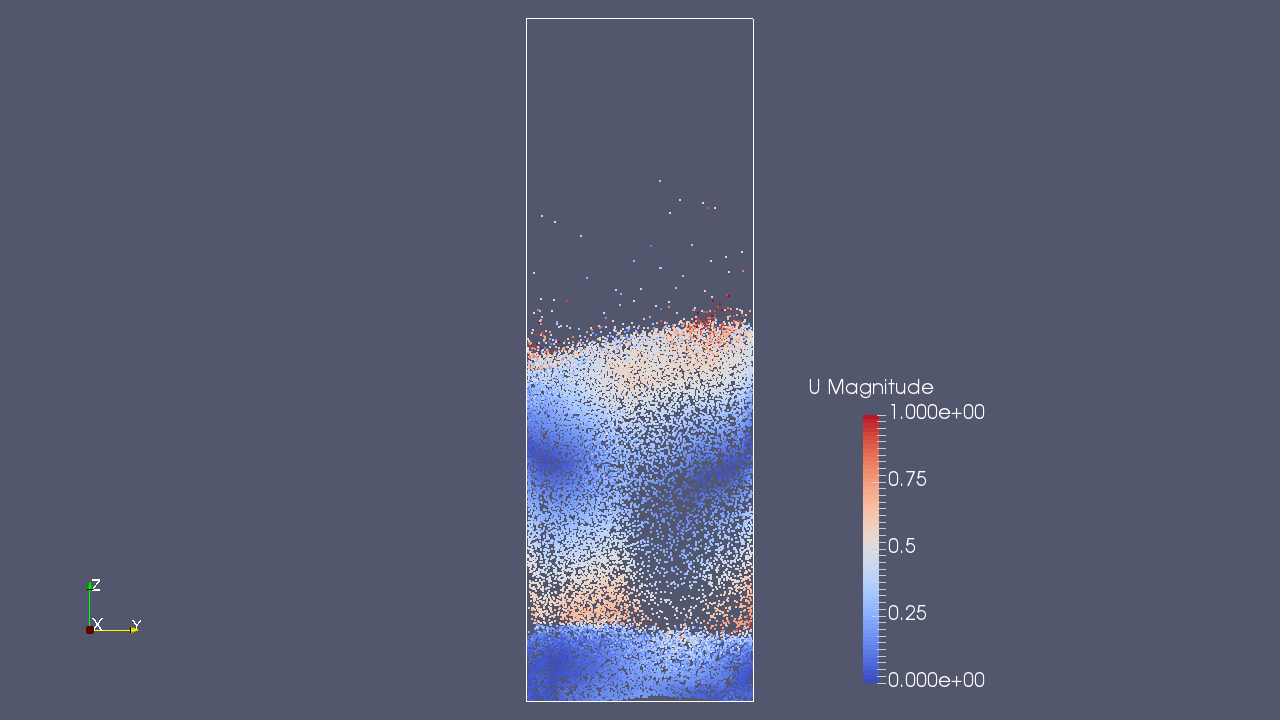Discrete particles and the velocity at 1 sec

## Commands

cp -r \$FOAM_TUTORIALS/lagrangian/MPPICFoam/Goldschmidt Goldschmidt
cd Goldschmidt

blockMesh
decomposePar
mpirun -np 5 MPPICFoam -parallel
reconstructParMesh
reconstructPar

paraFoam

## Calculation time

51 minutes 15.52 seconds *5 parallel, Inter(R) Core(TM) i7-2600 CPU @ 3.40GHz 3.40GHz